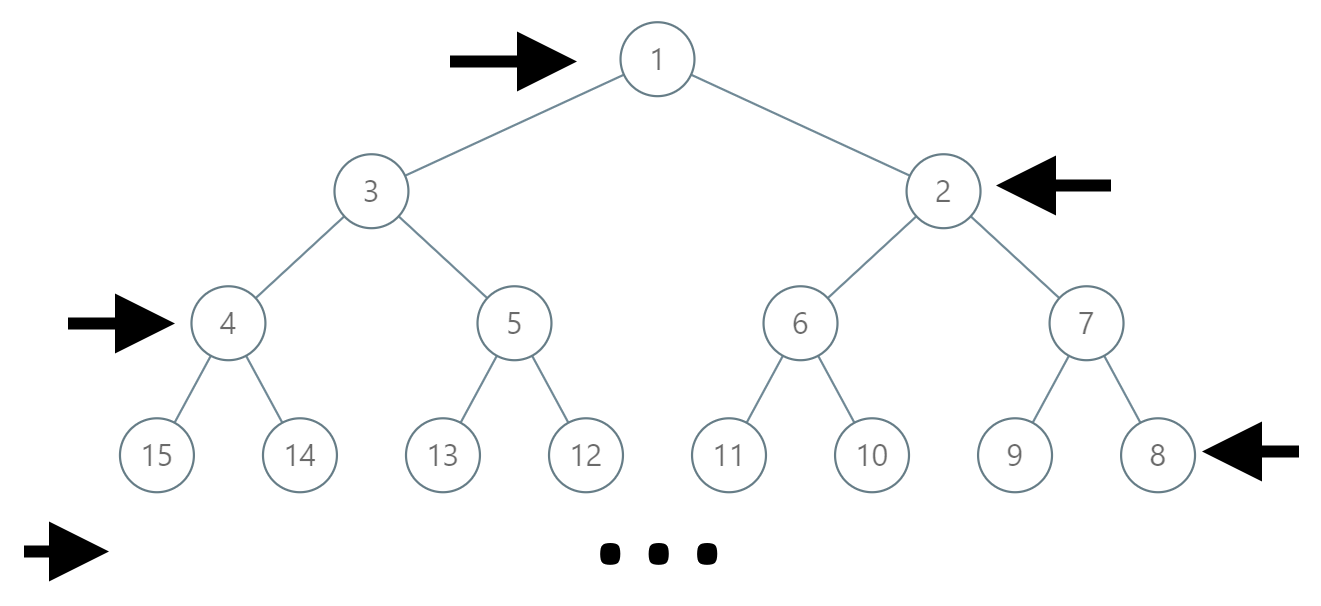## Algorithm

Problem Name: 1104. Path In Zigzag Labelled Binary Tree

In an infinite binary tree where every node has two children, the nodes are labelled in row order.

In the odd numbered rows (ie., the first, third, fifth,...), the labelling is left to right, while in the even numbered rows (second, fourth, sixth,...), the labelling is right to left.Given the `label` of a node in this tree, return the labels in the path from the root of the tree to the node with that `label`.

Example 1:

```Input: label = 14
Output: [1,3,4,14]
```

Example 2:

```Input: label = 26
Output: [1,2,6,10,26]
```

Constraints:

• `1 <= label <= 10^6`

## Code Examples

### #1 Code Example with Javascript Programming

```Code - Javascript Programming```

``````
const pathInZigZagTree = function(label) {
const res = [], { log2, floor, ceil } = Math
const level = floor(log2(label))
let compl = 2 ** (level + 1) - 1 + 2 ** level - label

while(label) {
res.push(label)
label = floor(label / 2)
compl = floor(compl / 2)
;[label, compl] = [compl, label]
}

res.reverse()

return res
};
``````
Copy The Code &

Input

cmd
label = 14

Output

cmd
[1,3,4,14]

### #2 Code Example with Python Programming

```Code - Python Programming```

``````
class Solution:
def pathInZigZagTree(self, label: int) -> List[int]:
res = [label]
while label != 1:
d = int(math.log(label) / math.log(2))
offset = int(2 ** (d + 1)) - label - 1
label = (int(2 ** d) + offset) // 2
res = [label] + res
return res
``````
Copy The Code &

Input

cmd
label = 14

Output

cmd
[1,3,4,14]

### #3 Code Example with C# Programming

```Code - C# Programming```

``````
using System.Collections.Generic;

namespace LeetCode
{
public class _1104_PathInZigzagLabelledBinaryTree
{
public IList PathInZigZagTree(int label)
{
int nodeCount = 1;
while (label >= nodeCount * 2)
nodeCount *= 2;

var result = new List();
while (label > 0)
{
var maxLevellable = nodeCount * 2 - 1;
var minLevellable = nodeCount;
var parent = (maxLevellable + minLevellable - label) / 2;

label = parent;
nodeCount /= 2;
}
result.Reverse();
return result;
}
}
}
``````
Copy The Code &

Input

cmd
label = 26

Output

cmd
[1,2,6,10,26]